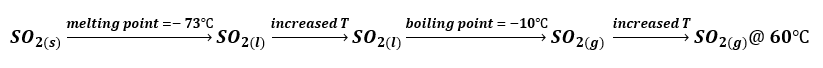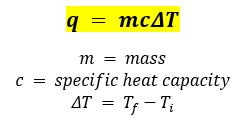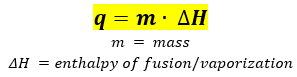# Problem: Sulfur dioxide is produced in enormous amounts for sulfuric acid production. It melts at −73°C and boils at −10.°C. Its ΔH°fus is 8.619 kJ/mol, and its ΔH°vap is 25.73 kJ/mol. The specific heat capacities of the liquid and gas are 0.995 J/g•K and 0.622 J/g•K, respectively. How much heat is required to convert 2.500 kg of solid SO2 at the melting point to a gas at 60.°C?

###### FREE Expert Solution

Four things happened to SO2 when it was converted from solid to gas as shown below:When calculating heat, we will use two equations:

1. Use the following equation for the steps where SO2 is in the same phase and increasing temperature:2. Use the following equation for phase changes:Calculate amount of heat required convert SO2(s) to SO2(l):

92% (223 ratings)###### Problem Details

Sulfur dioxide is produced in enormous amounts for sulfuric acid production. It melts at −73°C and boils at −10.°C. Its ΔH°fus is 8.619 kJ/mol, and its ΔH°vap is 25.73 kJ/mol. The specific heat capacities of the liquid and gas are 0.995 J/g•K and 0.622 J/g•K, respectively. How much heat is required to convert 2.500 kg of solid SO2 at the melting point to a gas at 60.°C?